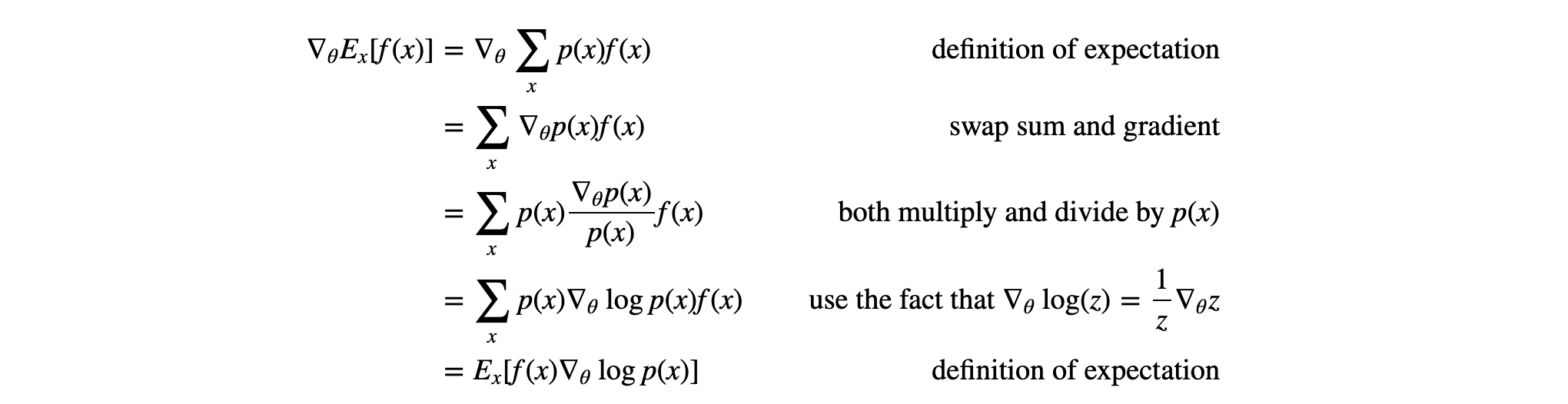# Deep Reinforcement Learning: Pong from Pixels学习笔记

Published:

Deep Reinforcement Learning: Pong from Pixels是一篇很好的强化学习入门博文。其中详细介绍了DRL一种常用的方法：Policy Gradient。原文链接：http://karpathy.github.io/2016/05/31/rl/

# 乒乓球游戏## 策略网络

h = np.dot(W1, x) # compute hidden layer neuron activations
h[h<0] = 0 # ReLU nonlinearity: threshold at zero
logp = np.dot(W2, h) # compute log probability of going up
p = 1.0 / (1.0 + np.exp(-logp)) # sigmoid function (gives probability of going up)


## 与有监督学习的关系

1. 有监督学习在得到logit并softmax之后，可以通过和真实标签计算交叉熵得到loss再计算梯度以及使用反向传播更新神经网络。然而在PG的学习过程中，我们并没有真实标签y，softmax之后得到的是产生每个action的概率，例如向上移动0.7，向下移动0.3。由于没有标签，只能通过对action的概率进行采样，采样到的action就是它的标签，所以PG的标签可以称为“假标签”。
2. 采样得到的action应用到环境中，然后通过环境给出的reward来计算梯度。所以PG的loss计算其实就是$A_i\log p(y_i\rvert x_i)$，$A_i$是在当前环境$x_i$下通过policy $p(x)$采样得到的行为$y_i$。因为一个step只能有一个action，所以softmax的其他项都是0（我们不可能在当前step计算每个action的$A_i$）。

## 折扣Reward

$R_t=\sum_{k=0}^\inf \gamma^kr_{t+k}=r_t + \gamma r_{t+1} + \gamma^2 r_{t+2} + \gamma^3 r_{t+3} +...$

## PG的数学推导

PG的loss，或者说是最终得分其实很简单，就是$E_{x\sim p(x\rvert\theta)}[f(x)]$，就是在当前policy $p(x)$下的得分$f(x)$的期望。所以我们需要计算的就是得分期望的梯度$\nabla_\theta E_{x\sim p(x\rvert\theta)}[f(x)]$。推导如下：# 一些思考

1. RL不接受任何规则输入，完全凭空通过试错去猜测游戏的模式。从这个角度来看，如果不跟人类说游戏规则，让人通过试错去猜测游戏玩法，那么RL会比人强很多。尤其是如果把屏幕上的像素位置全部打乱，人类根本无法通过视觉学会游戏规则，而RL可以轻易通过全连接的神经网络学会。
2. 人类带有大量的先验知识。例如球会反弹，弹出的角度与弹入的角度是一样的，方向键对应着球拍的移动，等等。然而RL什么都不知道，全凭摸索。
3. PG其实是一种暴力搜索的方法，尝试每个动作，然后把好的动作记下来。然而人类在学习新东西的时候，也会潜意识地将正确的行为变成肌肉记忆。例如骑车或游泳，一开始的时候会遍做遍想该怎么做，熟练（不停试错并且有正反馈）之后，则不需要思考，单靠肌肉记忆就可以完成。
4. 试错是RL学习的必要条件，然而不是人类学习的必要条件。还是由于先验知识，人类可以在不经历任何反馈的情况下，做出正确的行为。例如，人类不需要真实经历撞车几百次，才能学会如何避免撞车。先验知识决定了人类可以迅速将游戏规则（策略网络）高度抽象化（用一句话来描述游戏规则）。

Tags: